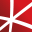# Top 20 NuGet numerics Packages

Foundational classes for financial, engineering, and scientific applications, including complex number classes, general vector and matrix classes, structured sparse matrix classes and factorizations, general sparse matrix classes and factorizations, general matrix decompositions, least squares solut...
The GPU-accelerated version of package CenterSpace.NMath. With a few minor exceptions, such as optional GPU configuration settings, the API is identical between CenterSpace.NMath.Premium and CenterSpace.NMath. If using at least .NET Framework 4.6.1 or .NET Core 2.0, we recommend using one of our NMa...
Foundational classes for financial, engineering, and scientific applications, including complex number classes, general vector and matrix classes, structured sparse matrix classes and factorizations, general sparse matrix classes and factorizations, general matrix decompositions, least squares solut...
Foundational classes for financial, engineering, and scientific applications, including complex number classes, general vector and matrix classes, structured sparse matrix classes and factorizations, general sparse matrix classes and factorizations, general matrix decompositions, least squares solut...
The Extreme Optimization Numerical Libraries for .NET are a set of libraries for numerical computing and data analysis. This is the main package that contains all the core functionality. For optimal performance, we strongly recommend also referencing one of the native packages based on Intel's Mat...
Bonsai Library providing methods and algorithms for common numerical computations.
Extreme Optimization Numerical Libraries for .NET Data Access Library for JSON.## NMath - Standard Library - OSX x64 (alpha)

Foundational classes for financial, engineering, and scientific applications, including complex number classes, general vector and matrix classes, structured sparse matrix classes and factorizations, general sparse matrix classes and factorizations, general matrix decompositions, least squares solut...
The Extreme Optimization Numerical Libraries for .NET are a set of libraries for numerical computing and data analysis. This package provides types specialized to single-precision, including complex numbers, as well as optimized implementations of single-precision linear algebra. Supports .NET Fra...
The Extreme Optimization Numerical Libraries for .NET are a set of libraries for numerical computing and data analysis. This package provides a complete implementation of linear algebra functionality for arbitrary numerical types. Supports .NET Framework 3.5, 4.0 and 4.6+, .NET Standard 1.3 and 2...
Class library implementing advanced mathematical algorithms, transforms, and time series manipulations. Implementations favour simplicity and correctness.
The Extreme Optimization Numerical Libraries for .NET are a set of libraries for numerical computing and data analysis. This package contains the single-precision mixed-mode native provider. This is the recommended native provider for the classic .NET Framework on Windows. Supports .NET Framework ...
The Extreme Optimization Numerical Libraries for .NET are a set of libraries for numerical computing and data analysis. This package contains the single-precision mixed-mode native provider. This is the recommended native provider for the classic .NET Framework on Windows. Supports .NET Framework ...
The Extreme Optimization Numerical Libraries for .NET are a set of libraries for numerical computing and data analysis. This package contains the mixed-mode native provider. This is the recommended native provider for the classic .NET Framework on Windows. Supports .NET Framework 4.0 and 4.6+ on W...
The Extreme Optimization Numerical Libraries for .NET are a set of libraries for numerical computing and data analysis. This package contains the mixed-mode native provider. This is the recommended native provider for the classic .NET Framework on Windows. Supports .NET Framework 3.5 on Windows.
Extreme Optimization Numerical Libraries for .NET P/Invoke MKL Provider for Windows.
Extreme Optimization Numerical Libraries for .NET Single-Precision P/Invoke MKL Provider for Windows.
Data Access Library for reading and writing files in commonly used formats including: R, Matlab, Text (CSV, delimited, fixed width), matrix market, stata. Part of the Extreme Optimization Numerical Libraries for .NET.
Extreme Optimization Numerical Libraries for .NET IronPython interface library
Extreme Optimization Numerical Libraries for .NET F# interface library.